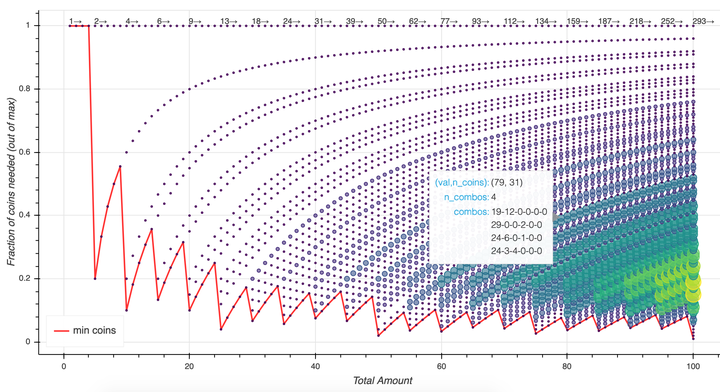# Dynamic Programming with Python dataclasses and joblibStarting in Python 3.7, the module dataclasses introduces a decorator that allows us to create immutable structures (like tuples) but with their own batteries-included methods. I wanted to give dataclasses a try with some non-trivial workloads. Having always been interested the coin change problem where the solution involves dynamic programming, this seemed to be a good test of dataclasses.

Instead of the classic coin change problem (finding the minimum number of coins that add up to a given amount), however, here I want to keep track of all possible combinations. The end result (at the bottom of this post) is an interactive plot to explore the results.

Note: You can get the original Jupyter notebook for this post here.

from dataclasses import dataclass, field
from joblib import Memory

from bokeh.io import output_notebook, show
from bokeh.plotting import figure

output_notebook()


Here we'll use joblib to cache the results, so we don't need to redo computations we already have completed:

cachedir = "/tmp/memo1"
memory = Memory(cachedir, verbose=0)
memory.clear()

WARNING:root:[Memory(location=/tmp/memo1/joblib)]: Flushing completely the cache


Now, let's define the universe of possible coins.

# coins = [1, 2, 5, 10, 20, 50, 100]  # European coins
# coins = [1, 5, 10, 25] # US Coins in wide circulation
coins = [1, 5, 10, 25, 50, 100] # All US Coins circulation


Here's where we create our dataclass—this will hold a specific collection of coins (the purse variable). We'll also define how to add coins from one purse to another (__add__) and set some helper methods allowing us to sort (__hash__), compare (__eq__, __le__, __lt__) and print (__repr__).

@dataclass
class change:
purse: list
value: int = field(init=False)
ncoins: int = field(init=False)
sort_index: int = field(init=False, repr=True)

def __post_init__(self):
self.value = sum(map(lambda x: x*x, zip(coins, self.purse)))
self.ncoins = sum(self.purse)
self.sort_index = self.ncoins

def n(self, coin):
return self.purse[coins.index(coin)]

return change([x+y for x,y in zip(self.purse,other.purse)])

def __eq__(self, other):
return tuple(self.purse) == tuple(other.purse)

def __le__(self, other):
return self.ncoins <= other.ncoins

def __lt__(self, other):
return self.ncoins < other.ncoins

def __hash__(self):
return hash(tuple(self.purse))

def __repr__(self):
return f"({self.value}) {dict(zip(coins,self.purse))}  {self.ncoins}"


Next we'll define the dynamic programming bit, which recursively looks for ways to make change, saving the results for a certain value, and reusing previously computed values.

empty_purse = *len(coins)

@memory.cache
def get_change(val):

if val < coins:
return [change(empty_purse)]
if val == coins:
purse = empty_purse.copy()
purse = 1
return [change(purse)]

final_list = []
for i, coin in enumerate(coins):
if val < coin:
continue

purse = empty_purse.copy()
purse[i] = 1
final_list += [prev + to_add for prev in get_change(val - coin)]

return list(set(final_list))

%time a = get_change(75)

CPU times: user 391 ms, sys: 74.3 ms, total: 466 ms
Wall time: 528 ms


That's pretty quick given all the possibilities (as we'll see). Let's compute all possibilities up to 100 cents.

max_n = 101
raw = [(x, sorted(get_change(x))) for x in range(1,max_n)]

points = []
uniques = []
min_coins = []
min_coins_scale = []

max_n = 0
for val, purse_list in raw:
ncoins = [x.ncoins for x in purse_list]
min_coins.append(min(ncoins))
min_coins_scale.append(min(ncoins)/val)
if val == 1:
uniques.append((val, len(ncoins)))
else:
if len(ncoins) != uniques[-1]:
uniques.append((val, len(ncoins)))

for nc in set(ncoins):
max_n = max(max_n, ncoins.count(nc))
combos = []
for x in [y for y in purse_list if y.ncoins == nc]:
combos.append("-".join([str(x) for x in x.purse]))
combos_str = "<br>".join(combos)
points.append((val, nc, ncoins.count(nc), combos_str))

print(max_n)

9


The value max_n is the most number of combinations for a certain fixed value size. For instance, if we want to make change for 97 cents with exactly 25 coins there are 7 different ways to do that:

PennyNickelDimeQuarter
17080
22003
17602
121201
12940
71800
17341

Now let's make an interactive plot:

from bokeh.models import ColumnDataSource,  LabelSet, Label
from bokeh.transform import linear_cmap
from bokeh.models import HoverTool

source = ColumnDataSource(data={
'x' : [x for x in points],
'y' : [x/(x) for x in points],
'y_nat': [x for x in points],
'n' : [max(2,20*x/max_n) for x in points],
'n_nat': [x for x in points],
'str': [x for x in points]
})

hover = HoverTool(point_policy='snap_to_data', line_policy='nearest')

hover.tooltips = [
("(val,n_coins)", "(@x, @y_nat)"),
("n_combos", "@n_nat"),
("combos", "@str{safe}")
]

source_label = ColumnDataSource(data=dict(x=[x for x in uniques],
y=[1 for x in uniques],
lab=[str(x) + '→' for x in uniques]))
labels = LabelSet(x='x', y='y', text='lab', level='glyph',
x_offset=-1, y_offset=0.01, source=source_label, render_mode='canvas', text_font_size="8pt")

p = figure(plot_width=975, plot_height=500)
p.xaxis.axis_label = 'Total Amount'
p.yaxis.axis_label = 'Fraction of coins needed (out of max)'

p.line([x for x in raw], min_coins_scale, line_width=2, color="red", alpha=0.8, legend_label="min coins")

p.circle('x', 'y', size='n', color=linear_cmap('n', 'Viridis256', 2, 20),
fill_alpha=0.6, source=source)

p.legend.location = "bottom_left"
p.legend.click_policy="hide"

p.tools.append(hover)

show(p)


This should be a dynamic plot – you can zoom in to see some of the interesting structures. Hover over circles to see the coin combinations. I color coded and sized the circles so you can see where there is a lot of non-unique combinations. For example, we can see that 30 cents is the lowest value where there is a non-unique number of coin combinations (you can get 30 with 6 coins in 2 different ways). The numbers at the top of the plot show the total number of unique solutions. Try this out on your own; for example, what differences do you see with the Euro cent combination?##### Joshua Bloom
###### Dept. Chair; Professor of Astronomy

Department Chair, Astrophysics Prof at UC Berkeley, former Wise.io cofounder (acquired by GE); Inventor; Dad, Tennis everything. Anti #TransparentMoon. Check out his group activities at ml4science.org.